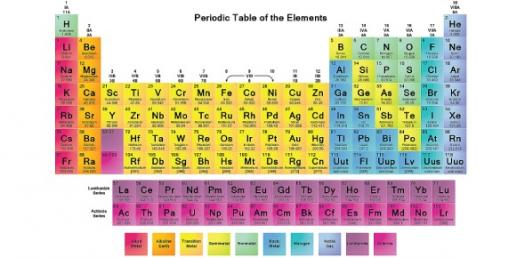# A Fundamentals Of Chemistry Quiz!

Approved & Edited by ProProfs Editorial Team
The editorial team at ProProfs Quizzes consists of a select group of subject experts, trivia writers, and quiz masters who have authored over 10,000 quizzes taken by more than 100 million users. This team includes our in-house seasoned quiz moderators and subject matter experts. Our editorial experts, spread across the world, are rigorously trained using our comprehensive guidelines to ensure that you receive the highest quality quizzes.
| By Nemoali
N
Nemoali
Community Contributor
Quizzes Created: 1 | Total Attempts: 223
Questions: 12 | Attempts: 234SettingsHere comes a basic, not too long Chemistry quiz for all those chemistry enthusiast out there. If you are the person whose playground is chemistry then I guess you would like to answer these questions. Have fun!

• 1.

• 2.

• 3.

• 4.

### Choose the correct answer for: The greatest number from the following:

• A.

10-9

• B.

10-2

• C.

10-3

B. 10-2
Explanation
The greatest number from the given options is 10-2. This can be determined by subtracting the numbers in each option from 10. When we subtract 9 from 10, we get 1. When we subtract 2 from 10, we get 8. When we subtract 3 from 10, we get 7. Therefore, the greatest number is 10-2, which is 8.

Rate this question:

• 5.

### From one dimension nano substance .............

• A.

Thin films.

• B.

Bucky ball.

• C.

Carbon nano tube.

A. Thin films.
Explanation
Thin films are a type of nano substance that can be formed by depositing a thin layer of material onto a substrate. This process allows for precise control over the thickness and properties of the film, making it useful in various applications such as electronics, optics, and coatings. Bucky balls and carbon nanotubes are also nano substances, but they are not specifically related to thin films. Therefore, the correct answer is thin films.

Rate this question:

• 6.

### Color of gold of nano scale is .............

• A.

Red.

• B.

Yellow.

• C.

Violet.

A. Red.
Explanation
The color of gold on a nano scale is red. This phenomenon is known as plasmon resonance, where the interaction of light with the electrons on the surface of the gold nanoparticles causes the absorption and scattering of certain wavelengths of light, resulting in a red color. This unique optical property of gold nanoparticles has various applications in fields such as medicine, electronics, and catalysis.

Rate this question:

• 7.

### Nano substances may have many different shapes    ________,

tubes , tubes , columns , wires , fibres , balls , thin films
• 8.

### Choose the correct balance for the following equation to be balanced chemical Equation?  N2 + H2  -----------  NH3

• A.

N2 + 3H2  -----------  2NH3

• B.

N2 + 4H2  -----------  2NH3

A. N2 + 3H2  -----------  2NH3
Explanation
The balanced chemical equation for the reaction between nitrogen gas (N2) and hydrogen gas (H2) to form ammonia (NH3) is N2 + 3H2 → 2NH3. This is because the number of nitrogen atoms on both sides of the equation is the same (1 nitrogen atom), and the number of hydrogen atoms is also the same (6 hydrogen atoms). Therefore, this equation satisfies the law of conservation of mass, which states that matter cannot be created or destroyed in a chemical reaction.

Rate this question:

• 9.

### Fe3O4 + O2 -------- Fe2O3

• A.

Fe3O4 + 2O2 -------- Fe2O3

• B.

2Fe3O4 + 1/2 O2 -------- 3Fe2O3

B. 2Fe3O4 + 1/2 O2 -------- 3Fe2O3
Explanation
The balanced equation shows the reaction between Fe3O4 and O2 to form Fe2O3. The coefficients in front of the reactants and products indicate the number of moles of each substance involved in the reaction. In this case, the equation is balanced when there are 2 moles of Fe3O4 reacting with 1/2 mole of O2 to produce 3 moles of Fe2O3. This balanced equation follows the law of conservation of mass, ensuring that the same number of atoms of each element are present on both sides of the equation.

Rate this question:

• 10.

### Solve the following Equation in Ionic form :  NH4Cl (aq) ---------- ................................

• A.

NH4(aq)    +  Cl(aq)

• B.

NH4(aq) -   +  Cl+ (aq)

A. NH4(aq)    +  Cl(aq)
• 11.

### Mention the suitable name of process for the following :  a-H+ (aq)    +     OH (aq) - -------------------- H2O (l)

• A.

Melting.

• B.

Neutralization.

• C.

Dissolving.

• D.

None of them.